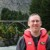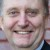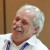# DPMO to a Sigma Level

Six Sigma – iSixSigma Forums General Forums Tools & Templates DPMO to a Sigma Level

Viewing 6 posts - 1 through 6 (of 6 total)
• Author
Posts
• #55842Alf M
Guest

Hi everyone,

I was wondering if someone could help me understand the question below, the old fashioned way (Paper, Pencil & Calculator).

The DPMO for a process is 860. What is the approximate six sigma level of the process?

6 σ = 0.8406 + √29.37 – 2.221 x ln(860)
=0.8406 + √29.37 -15.01
=0.8406 + 3.7895 = 4.63

The result is 4.63

Question: Where 15.01 come from? What calculation ?

I know the 3.785 come from 29.37 -15.01 = 14.36 = √ 3.7895, but where 15.01 come from? Which calculation?

Thanks in advance I will very much appreciate the help.
Alf

0
#201883Chris Seider
Participant

Have you considered @normsdist and @normsinv with excel. There are normal distribution charts that exist to do it with paper/pencil also.

You are using the normal distribution for converting ppm defective or DPMO to sigma level. There are previous posts on this also.

Hope this helps.

0
#201897Guest

The correct calculation formula is:

Process Sigma = 0.8406 + SQRT(29.37 – 2.221 * (ln(DPMO))) =
0.8406 + SQRT (29.37 – 2.221 * 6.76) = 0,8406 + SQRT (29.37 – 14.9396) = 0.8406 + SQRT (14.4304) = 0.8406 + 3.7987 = 4.6393

There is not any 15.01 value.

0
#201904Andrew Parr
Participant

I’m impressed that people are still wanting to understand the calculation and how to get the correct answer with pencil and paper rather than just put the numbers into the Six Sigma Calculator on here.

0
#202739C.J. Kisabeth
Guest

The 15.01 is coming from 0.8406 + SQRT (29.37 – 2.221 * 6.76), where 2.221 * 6.76 = 15.01

0
#202769Mike Carnell
Participant

@alf M I have attached a chart I got from Gary Cone at GPS consultants

0
Viewing 6 posts - 1 through 6 (of 6 total)

You must be logged in to reply to this topic.